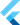# Methods: static, private, etc

on Sunday, 19th of July, 2020

You have several options when defining methods on classes. First, let's look at basic examples of each.

``````class Microwave {
// basic method
void startCooking (){}

// private method
// this cannot be called from outside the class itself
// it is not exposed to _instances_ of the class
void _startTimer() {}

// a static method isn't tied to an instance
// rather, it's logically tied to a class.
// static methods are compile-time constant,
static bool compareWatts(Microwave a, Microwave b) {
if (a.power > b.power) return -1;
if (b.power > a.power) return 1;

// else they're the same
return 0;
}
}``````
``````class Microwave {
final int power;

Microwave(this.power);

void startCooking() {
_startTimer();
print('starting cooking!')
}

void _startTimer() {
print('starting timer!');
}

static int compareWatts(Microwave a, Microwave b) {
if (a.power > b.power) return -1;
if (b.power > a.power) return 1;

// else they're the same
return 0;
}
}

void main() {
var averageMicrowave = Microwave(1100);
var miniMicrowave = Microwave(800);
var superMicrowave = Microwave(1500);

// calling a basic instance method
averageMicrowave.startCooking();

// calling a static method
// which you must call on the class, rather than the instance
print(Microwave.compareWatts(averageMicrowave, superMicrowave));
}``````